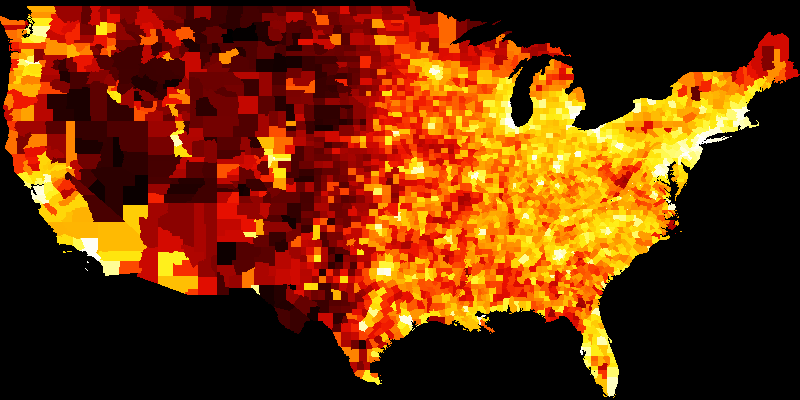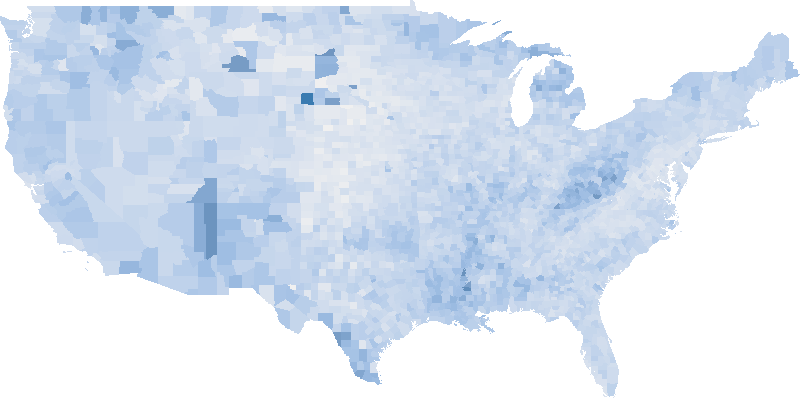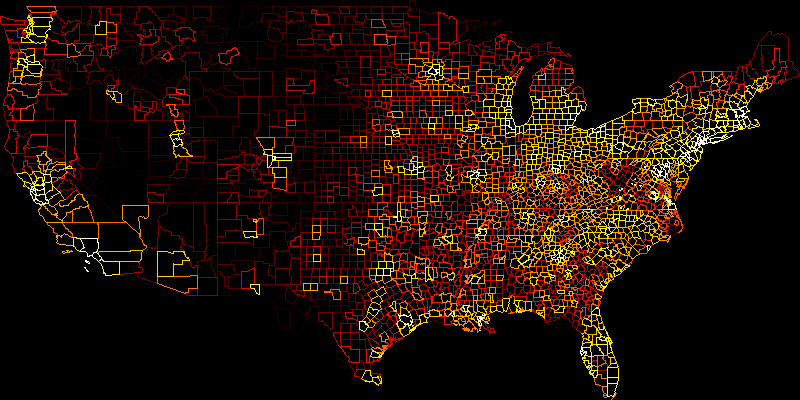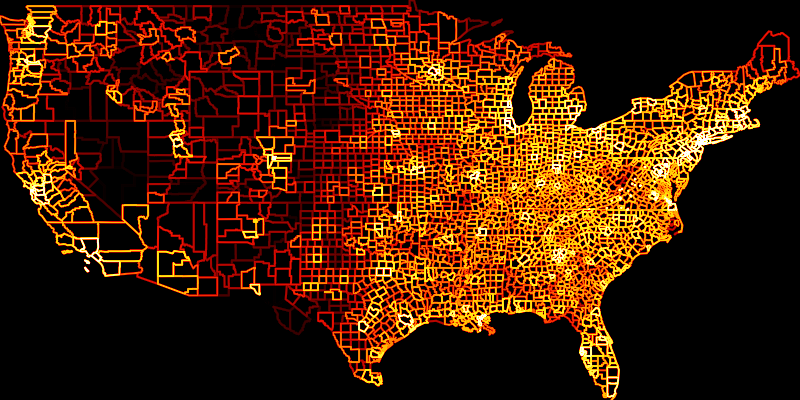# Geopandas#

From version 0.16 onwards Datashader supports rendering GeoPandas `GeoDataFrame`s directly rather than having to convert them to SpatialPandas first.

Here is a demonstration using the “geoda.natregimes” dataset from `geodatasets`, which includes data on US counties.

```import colorcet as cc
import geopandas
from geodatasets import get_path
```

First load the GeoPandas `GeoDataFrame`. The first time this is called will download and cache the dataset, and subsequent calls will be faster as they will use the cached dataset.

```df = geopandas.read_file(get_path("geoda.natregimes"))
```
```Downloading file 'natregimes.zip' from 'https://geodacenter.github.io/data-and-lab//data/natregimes.zip' to '/home/runner/.cache/geodatasets'.
```
REGIONS NOSOUTH POLY_ID NAME STATE_NAME STATE_FIPS CNTY_FIPS FIPS STFIPS COFIPS ... GI59 GI69 GI79 GI89 FH60 FH70 FH80 FH90 West geometry
0 1.0 1.0 1 Lake of the Woods Minnesota 27 077 27077 27 77 ... 0.285235 0.372336 0.342104 0.336455 11.279621 5.4 5.663881 9.515860 0 POLYGON ((-95.34258 48.54670, -95.34081 48.715...
1 2.0 1.0 2 Ferry Washington 53 019 53019 53 19 ... 0.256158 0.360665 0.361928 0.360640 10.053476 2.6 10.079576 11.397059 1 POLYGON ((-118.85050 47.94969, -118.84732 48.4...
2 2.0 1.0 3 Stevens Washington 53 065 53065 53 65 ... 0.283999 0.394083 0.357566 0.369942 9.258437 5.6 6.812127 10.352015 1 POLYGON ((-117.43777 48.04422, -117.54113 48.0...
3 2.0 1.0 4 Okanogan Washington 53 047 53047 53 47 ... 0.258540 0.371218 0.381240 0.394519 9.039900 8.1 10.084926 12.840340 1 POLYGON ((-118.97096 47.93928, -118.97293 47.9...
4 2.0 1.0 5 Pend Oreille Washington 53 051 53051 53 51 ... 0.243263 0.365614 0.358706 0.387848 8.243930 4.1 7.557643 10.313002 1 POLYGON ((-117.43750 49.00000, -117.03098 49.0...

5 rows × 74 columns

The geometry type of this `GeoDataFrame` is POLYGON, and there are many columns. Columns that we will use are “DNL90” (log of population density in 1990) and “UE90” (unemployment rate in 1990).

## Population#

To view 1990 population data using Datashader, first create a canvas to render to of an appropriate size.

```canvas = ds.Canvas(plot_width=800, plot_height=400)
```

The polygons are rasterized to the canvas using the `Canvas.polygons` method. This takes the source dataframe and name of the geometry column, plus an aggregator. Here we aggregate using the maximum of the population density column so that if there are multiple polygons touching a particular pixel it selects the maximum population density of those polygons.

```agg = canvas.polygons(df, geometry="geometry", agg=ds.max("DNL90"))
```

Now we shade the aggregation using Colorcet’s `fire` colormap using histogram equalization so that the colors are applied nonlinearly for most even use of the colormap.

```im = tf.shade(agg, cmap=cc.fire, how="eq_hist")
tf.set_background(im, "black")
```Counties with the lowest population density are rendered in black and dark red, and those with the highest population density are rendered in yellow and white.

## Unemployment#

The “UE90” column contains unemployment percentage per county in 1990.

```df["UE90"], df["UE90"].min(), df["UE90"].max()
```
```(0        3.894790
1       16.811594
2       10.700794
3       10.203544
4       14.991023
...
3080    10.910351
3081    11.002271
3082     4.102888
3083     3.353981
3084     5.488553
Name: UE90, Length: 3085, dtype: float64,
0.0,
30.534082923)
```

We can see from the `min` and `max` values that unemployment goes from zero to just over 30%.

To rasterize this using Datashader it is recommended to use a monochromatic colormap such as `colorcet.blues` and apply this linearly.

```agg = canvas.polygons(df, geometry="geometry", agg=ds.max("UE90"))
tf.set_background(im, "white")
```## Lines#

Datashader can also render GeoPandas `GeoDataFrame`s as lines rather than polygons. Use the same code as in the population example above but replace `Canvas.polygons()` with `Canvas.line()` instead.

```agg = canvas.line(df, geometry="geometry", agg=ds.max("DNL90"))
tf.set_background(im, "black")
```Lines can be rendered with antialiasing. Here is the previous example with an antialiased line width of 2 pixels.

```agg = canvas.line(df, geometry="geometry", agg=ds.max("DNL90"), line_width=2)
tf.set_background(im, "black")
```## Geometry type support#

The following table shows which geometry types are supported by which Datashader `Canvas` functions.

Canvas function

Supported geometry types

`Canvas.line`

LineString, MultiLineString, MultiPolygon, Polygon

`Canvas.point`

MultiPoint, Point

`Canvas.polygons`

MultiPolygon, Polygon

## GeoPandas or SpatialPandas?#

Datashader supports the same line, point and polygon rendering using GeoPandas and SpatialPandas, and produces the same output using either. They work in different ways such that SpatialPandas is usually faster for viewing large datasets and GeoPandas faster when zooming into a small region of a large dataset. The GeoPandas approach is more convenient if you already have your data in GeoPandas format and do not want the overhead of converting to SpatialPandas.

This web page was generated from a Jupyter notebook and not all interactivity will work on this website. Right click to download and run locally for full Python-backed interactivity.